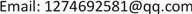1. 引言

2. 车道线检测方法2.1. 总体框架

1) 感兴趣区域截取。车道线主要位于图像的下半部分，为兼顾准确度与实时性，本文提取图像底部的2/3区域为感兴趣区域。

2) 灰度化。人眼对绿色敏感度最高，对蓝色敏感度最低，对彩色图像中的R、G、B三个分量加权平均得到灰度图像，其灰度值计算如公式(1)：

Y = 0.3 R + 0.59 G + 0.11 B (1)

3) 方向可调滤波。根据统计的左右车道线方向角均值和方差  ，确定方向可调滤波器的输入角并进行边缘增强。方向可调滤波器为二维高斯函数的一阶导数的线性组合。

4) 图像二值化。利用Otsu  阈值对图像进行二值化。

5) 边缘检测。利用Canny算子进行图像边缘检测。

6) 概率霍夫变换拟合车道线。根据统计的左右车道线方向角均值和方差，对左右车道线极角范围进行限定，并利用概率霍夫变换对车道线进行拟合。

2.2. 基于方向可调滤波的车道线检测

G ( x , y ) = exp ( − ( x 2 + y 2 ) ) (2)

G 1 0 ˚ = ∂ ∂ x exp ( − ( x 2 + y 2 ) ) = − 2 x exp ( − ( x 2 + y 2 ) ) (3)

G 1 90 ˚ = ∂ ∂ y exp ( − ( x 2 + y 2 ) ) = − 2 y exp ( − ( x 2 + y 2 ) ) (4)

G 1 θ = G 1 0 ˚ cos θ + G 1 90 ˚ sin θ (5)

I θ = f * G 1 θ = f * G 1 0 ˚ cos θ + G 1 90 ˚ sin θ = cos θ + sin θ (6)

2.3. 基于概率霍夫变换的车道线拟合

1) 建立并初始化一个累加器 A ( ρ , θ ) ，将车道线检测获取的边缘图像前景点放入待选边缘点集S；

2) 集合S若为空则算法结束；否则从集合S中随机取一点 ( x , y ) 投射到参数空间，并从S中去掉该点，然后在 θ l 、 θ r 范围内计算相应的 ρ 值，并更新对应的累加器 A ( ρ , θ ) ；

3) 对 A ( ρ , θ ) 与预定的阈值比较，若大于阈值且满足最小线段长度，则执行步骤4)，否则回到步骤2)；

4) 取出该直线，完成一条直线的拟合。

5) 删除S中位于直线上的所有点，并将累加器清零，再继续执行步骤2)。

3. 实验结果与对比3.1. 车道线检测实验

3.2. 与其它方法的对比

4. 结论